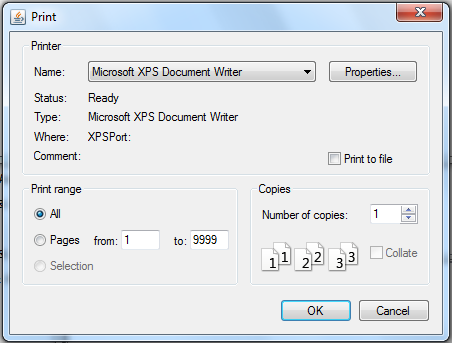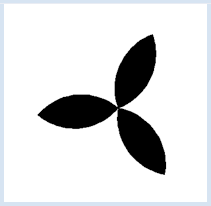deutsch     english    français     Print

## 2.12 PRINTING### INTRODUCTION

 To achieve higher precision you can draw graphics on a printer since printers usually have a much higher resolution, for example 1200x1200 dpi, where a typical screen resolution is around 100 dpi. The printing of turtle graphics works in a way where graphic operations are printed onto paper as high-resolution vector graphics instead of being displayed on the screen. For this, you can define a function with any name that contains all of the instructions for creating the image. When it is called directly, the image appears on the screen. To print, call printerPlot() with the function name. A printer dialog opens where you can select the printer and set its properties. You can then also print on virtual printers to create a graphic file in a high resolution format (for example TIFF or EPS).PROGRAMMING CONCEPTS: High resolution graphics

### A NON-FIRE-BREATHING DRAGON

 To show a fun example of high-resolution printing, let the turtle draw a complicated picture called the dragon curve. Although you could also make this curve by folding a strip of paper, it is much easier with computer graphics. However, the implementation of the folding instructions in an algorithm is not entirely trivial [more... You can orient yourself on the Internet, what exactly is a dragon curve].Since we are dealing with printing here, we simply use a pre-defined function to draw the curve, figure(s, n, flag). At least you can see that the curve is defined recursively and calls itself twice, although with a lower order n-1. Additionally, the function uses a parameter flag that can be 1 or -1 and that determines the drawing direction.

To print the image, draw it in the function doIt(). This function cannot have parameters. When you call doIt() the drawing appears on the screen, but when you pass the name doIt to the printerPlot() command the drawing is printed (without showing the turtle).

```from gturtle import *

def drawFigure():
repeat 3:
fillToPoint(0, 0)
rightArc(100, 90)
right(90)
rightArc(100, 90)
right(90)
left(120)

makeTurtle()
hideTurtle()
setPenColor("black")

while True:
drawFigure()
delay(100)
clear()
right(20)
```
Highlight program code (Ctrl+C copy, Ctrl+V paste)

### MEMO

 You have to position the drawing on the sheet properly by using setPos(). Depending on the size of the turtle window and the printer being used, this position can change. When calling printerPlot() you can also specify a scaling factor k in printerPlot(doIt, k). The image enlarges when k > 1 and shrinks when k < 1.

### EXERCISES

1.

 Joshua Goldstein suggests using pairs of move/turn commands to create nice pictures. A step consists of the commands:    forward(s)    right(a) Draw and print the following Goldstein figures: a. 31 steps where s = 300,  a = 151° b. 142 steps where s = 400,  a = 159.72° You must provide the positioning yourself!

2.

 A step can also consist of two move/turn pairs. Draw and print the following Goldstein figure: 37 steps where s = 77, a = 140.86° and s = 310, a = 112°

3.

 Draw and print the Goldstein figure with three move/turn pairs: 47 steps where s = 15.4, a = 140.86 ° and s = 62, a = 112° and s= 57.2, a = 130°